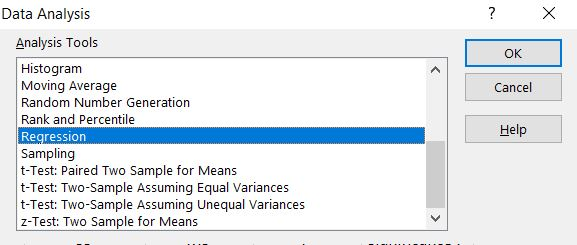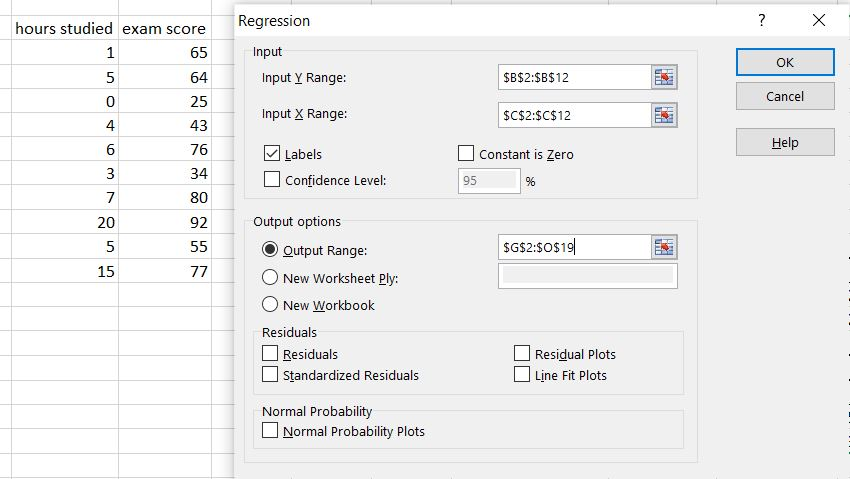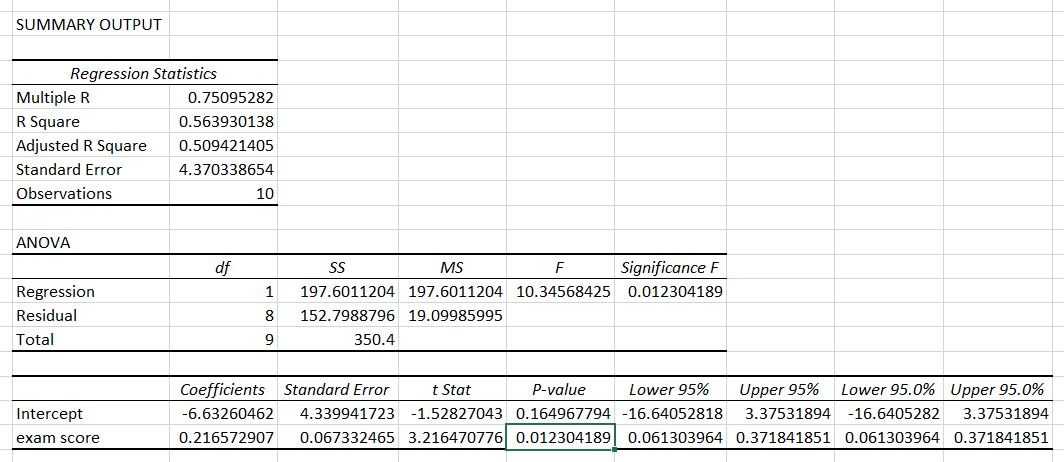#### How to calculate p value in Excel

In this Excel tutorial you will teach yourself how to calculate p value in Excel.

## What is a P-Value?

A p-value is a statistical measure that quantifies the probability of obtaining a result as extreme as, or even more extreme than, the one observed in your study if the null hypothesis were true. In essence, it tells you how likely it is that the observed results are due to random chance.

## How to calculate p value in Excel?

To calculate p-value use Data Analysis Toolpak add-in.

Here’s how to add the analysis toolpak on Excel.

First prepare your data. I have two sets of value how many hours students studied to the exam and their exam scores.

Click Data Analysis button and choose Regression.Put input values. In my example Y Range are hours studied and X Range is for exam scores.

As and Output Range choose where would you like to put results of Regression analysis in your sheet.Here there are results of regression analysis.P value of exam score is exactly 0.012304189.

You got also other values like Standard Error and Anova test.

## How to interpret p value?

The interpretation of the p-value is crucial in assessing the significance of your findings. Here’s a general guideline.

See the p value:

• Significant: <=0.05
• Marginally significant: <=0.1
• Insignificant: >0.1

In my example it is significant because 0.012304189 is less than 0.05. It was worth to study hard to get better exam score.

While p-values indicate the statistical significance of results, they don’t provide information about the practical significance or effect size of an observed relationship. It’s crucial to consider effect size measures (e.g., Cohen’s d, correlation coefficients) alongside p-values to assess the practical importance of your findings. Excel can calculate some effect size measures, but these typically require additional formulas or custom functions.